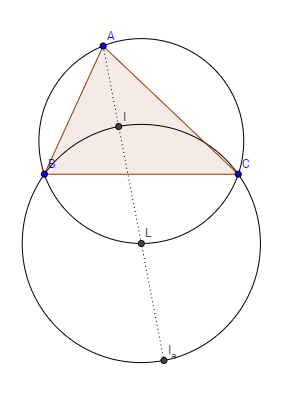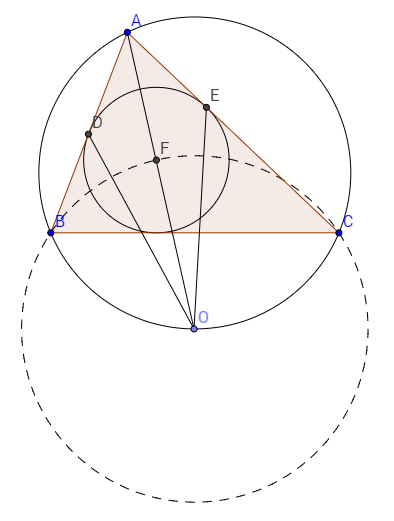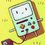# A Nice Problem Trivialized By a Single Lemma!

(CGMO 2012) The incircle of a triangle ABC is tangent to sides AB and AC at D and E respectively, and O is the circumcenter of triangle BCI where $$I$$ denotes the incenter of $$\bigtriangleup ABC$$. Prove that $$\angle ODB = \angle OEC$$

$\textbf{Proof}$

We will first prove this lemma.

$\textbf{Lemma}$ Let $ABC$ be a triangle with incenter $I$ , A-excenter $I_a$, and denote the midpoint of arc $BC$ to be $L$, then points $B, I, C, I_a$ are concyclic with $L$ as the circle center.

(We will denote angle $A$ , $B$, $C$, as $\alpha, \beta,$ and $\gamma$, respectively.)

Also note that $\alpha + \beta + \gamma = 180$Proof: First notice that $L$ is the intersection of the angle bisector of $A$ and perpendicular bisector of $BC$.

First we prove that $LB = LI = LC$.

Since the perpendicular of $L$ to $BC$ is the perpendicular bisector of $BC$, we know that $LB = LC$.

Because $BI, AI$ are angle bisectors, we know that $\angle IAB = \frac{\alpha}{2}$ and $\angle ABI= \angle IBC = \frac{\beta}{2}$. By Exterior Angle Theorem, we know that $\angle BIL = \frac{\alpha + \beta}{2}$. If we prove that $\angle IBL = \frac{\alpha + \beta}{2}$ we have proved the segments are equal.

Notice that $\angle CBL = \angle LAC = \frac{\alpha}{2}$ because they substend the same arc. Therefore this implies that $\angle IBL = \angle IBC + \angle CBL = \frac{\alpha+\beta}{2} = \angle BIL$. Thus, by isosceles triangles we get that $LB = LI = LC$. This tells us that $B, I, C$ are concyclic with $L$ as a center.

Now, we must prove that $I_a$ also lies on this circle.

Recall that the $I_a$ is the intersection of the exterior-angle bisectors of $B$ and $C$. Therefore if you extend $AB$ and $AC$ and construct the angle bisector of the exterior angle, it intersects at $I_a$.

Denote $M$ as a point on ray $AB$ past B.

By Exterior Angle Theorem, we have that $\angle MBC = \alpha + \gamma$.

Since $BI_a$ is the angle bisector, we have that $\angle I_aBC = \frac{\alpha + \gamma }{2}$ . Since $\angle LBC = \frac{\alpha}{2}$, this implies that $\angle I_aBL = \frac{\gamma}{2}$.

We proved that $\angle LBI = \angle LIB = \frac{\alpha + \beta}{2} \implies \angle BLI = \gamma$ .

Again by exterior angle theorem, since $\angle LBI_a = \frac{\gamma}{2}$, we have that $\angle BI_aL = \frac{\gamma}{2}$ which implies that $\bigtriangleup BLI_a$ is isoscles which implies that $BL = LI_a \implies BL = LI_a = IL = LC$. Therefore $I_a$ must be concyclic with $B, I, C$ because it's radius to the center, $L$, is the same. Therefore, we have proved the lemma.

Okay good. Now let's have fun.Restatement: The incircle of a triangle ABC is tangent to sides AB and AC at D and E respectively, and O is the circumcenter of triangle BCF where $F$ denotes the incenter of $\bigtriangleup ABC$. Prove that $\angle ODB = \angle OEC$

By the lemma, we know that the circumcenter, $O$, of $\bigtriangleup BFC$ is actually the midpoint of arc BC of the circumcircle of $\bigtriangleup ABC$.

To prove $\angle ODB = \angle OEC$, it suffices to prove that $\angle ADO = \angle AEO$.

Since $O$ is on the angle bisector, we know that $\angle DAO = \angle OAE$. We also know that $AD = AE$ because they are both tangents to the incircle. Additionally, $\bigtriangleup DAO$ and $\bigtriangleup AOE$ share side $AO$. Therefore, by $SAS$, we have that $\bigtriangleup ADO \cong \bigtriangleup AEO$.

This implies that $\angle ADO = \angle AEO \implies \angle ODB = \angle OEC$ and we are done.Note by Alan Yan
5 years, 9 months ago

This discussion board is a place to discuss our Daily Challenges and the math and science related to those challenges. Explanations are more than just a solution — they should explain the steps and thinking strategies that you used to obtain the solution. Comments should further the discussion of math and science.

When posting on Brilliant:

• Use the emojis to react to an explanation, whether you're congratulating a job well done , or just really confused .
• Ask specific questions about the challenge or the steps in somebody's explanation. Well-posed questions can add a lot to the discussion, but posting "I don't understand!" doesn't help anyone.
• Try to contribute something new to the discussion, whether it is an extension, generalization or other idea related to the challenge.
• Stay on topic — we're all here to learn more about math and science, not to hear about your favorite get-rich-quick scheme or current world events.

MarkdownAppears as
*italics* or _italics_ italics
**bold** or __bold__ bold
- bulleted- list
• bulleted
• list
1. numbered2. list
1. numbered
2. list
Note: you must add a full line of space before and after lists for them to show up correctly
paragraph 1paragraph 2

paragraph 1

paragraph 2

[example link](https://brilliant.org)example link
> This is a quote
This is a quote
    # I indented these lines
# 4 spaces, and now they show
# up as a code block.

print "hello world"
# I indented these lines
# 4 spaces, and now they show
# up as a code block.

print "hello world"
MathAppears as
Remember to wrap math in $$ ... $$ or $ ... $ to ensure proper formatting.
2 \times 3 $2 \times 3$
2^{34} $2^{34}$
a_{i-1} $a_{i-1}$
\frac{2}{3} $\frac{2}{3}$
\sqrt{2} $\sqrt{2}$
\sum_{i=1}^3 $\sum_{i=1}^3$
\sin \theta $\sin \theta$
\boxed{123} $\boxed{123}$

## Comments

Sort by:

Top Newest

First, let's clarify that point I and point F are the one and the same, which is the incenter of triangle ABC. It seems to me that it's immediately obvious that the quadrilateral AEOD is a kite, so that angles $\angle$ODA = $\angle$OEA, and therefore angles $\angle$ODB = $\angle$OEC? Am I missing something here?

- 5 years, 9 months ago

Log in to reply

It is true that AEOD is a kite, but can you prove it?

- 5 years, 9 months ago

Log in to reply

If, given two points on a circle, the tangents through are drawn so that they intersect at a 3rd point, the distances from the 3rd point to the first two points are the same. Any point on the line that passes through this 3rd point and the center of the circle forms the 4th point of the kite, by reason of symmetry.

- 5 years, 9 months ago

Log in to reply

Yes...that works. But note both arguments rely on the fact that O lies on the angle bisector of A, and that was the main concept I was trying to convey.

- 5 years, 9 months ago

Log in to reply

I think by using the Lemma you've discussed, a harder geometry problem probably can be devised. Let me think on it for a while on how that can be done.

- 5 years, 9 months ago

Log in to reply

I agree. Please do so :)

- 5 years, 9 months ago

Log in to reply

×

Problem Loading...

Note Loading...

Set Loading...